Скачать презентацию A Power Point Tutorial to Accompany macroeconomics

aab6dc98d673efc5cce0cded98107a24.ppt

• Количество слайдов: 22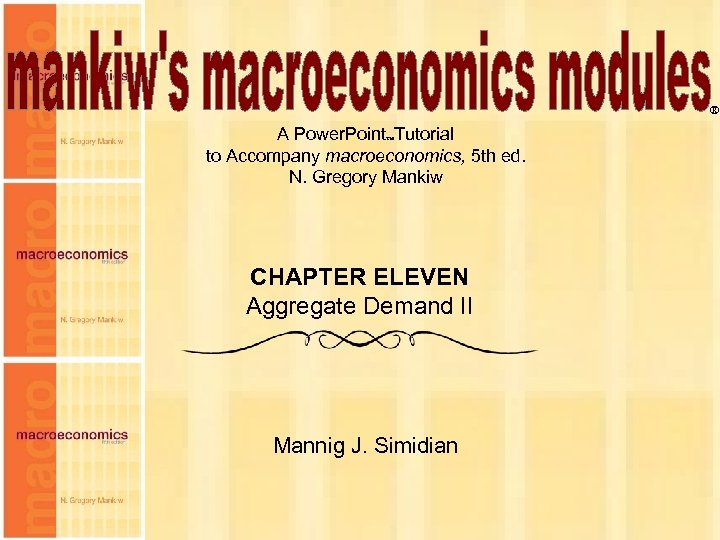® A Power. Point Tutorial to Accompany macroeconomics, 5 th ed. N. Gregory Mankiw CHAPTER ELEVEN Aggregate Demand II Mannig J. Simidian Chapter Eleven 1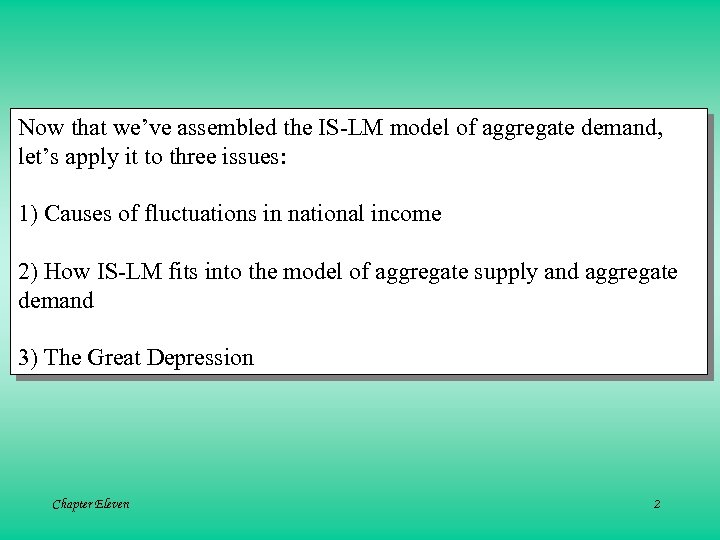Now that we’ve assembled the IS-LM model of aggregate demand, let’s apply it to three issues: 1) Causes of fluctuations in national income 2) How IS-LM fits into the model of aggregate supply and aggregate demand 3) The Great Depression Chapter Eleven 2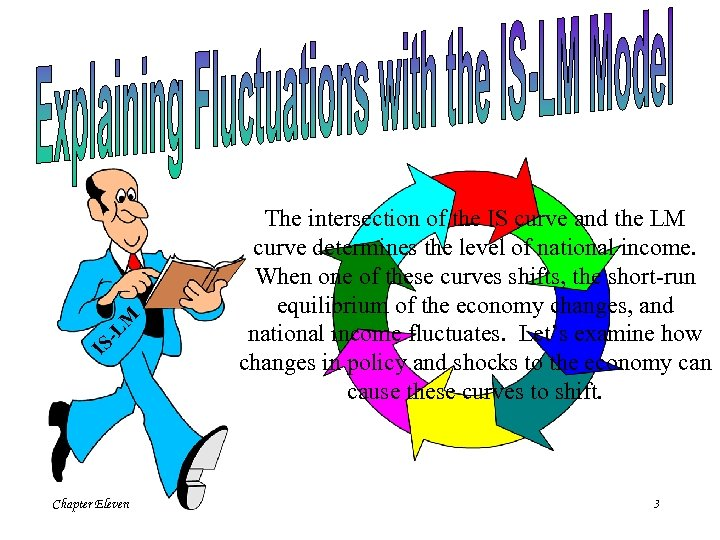-L M IS Chapter Eleven The intersection of the IS curve and the LM curve determines the level of national income. When one of these curves shifts, the short-run equilibrium of the economy changes, and national income fluctuates. Let’s examine how changes in policy and shocks to the economy can cause these curves to shift. 3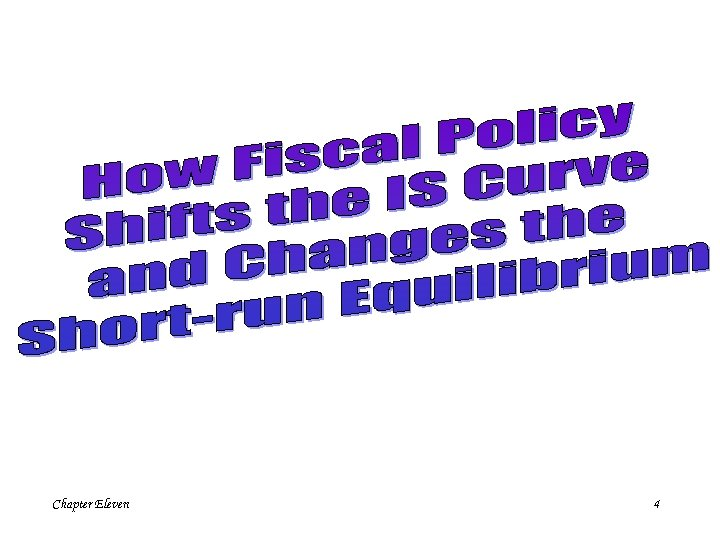Chapter Eleven 4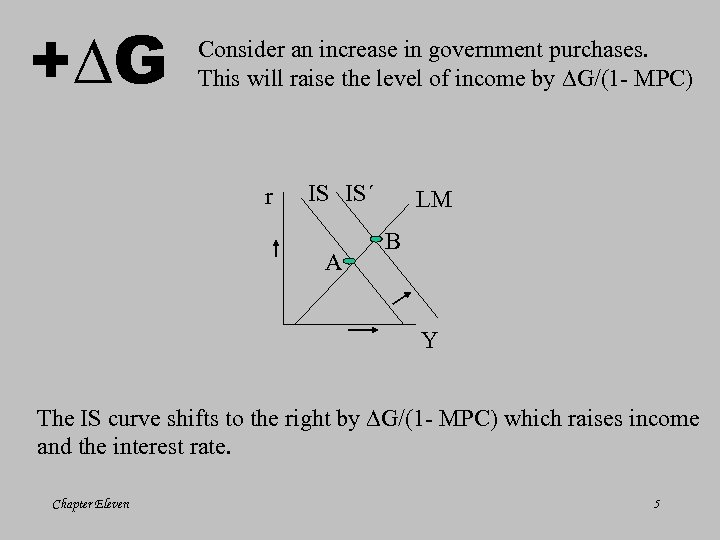+ G Consider an increase in government purchases. This will raise the level of income by G/(1 - MPC) r IS IS´ A LM B Y The IS curve shifts to the right by G/(1 - MPC) which raises income and the interest rate. Chapter Eleven 5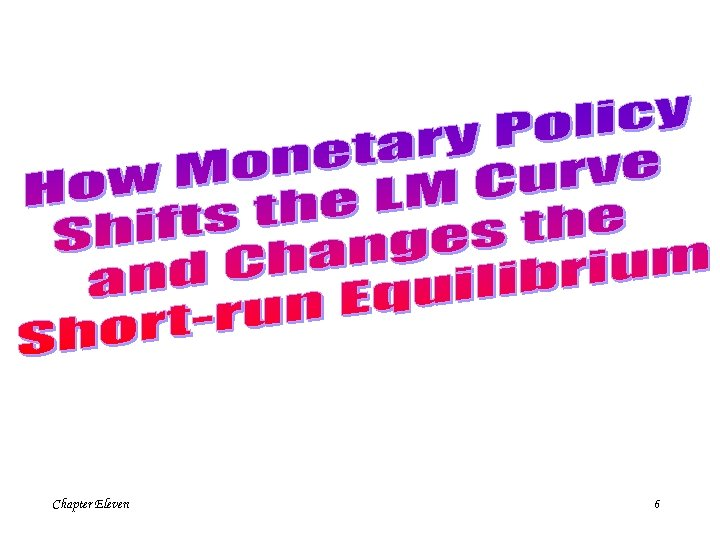Chapter Eleven 6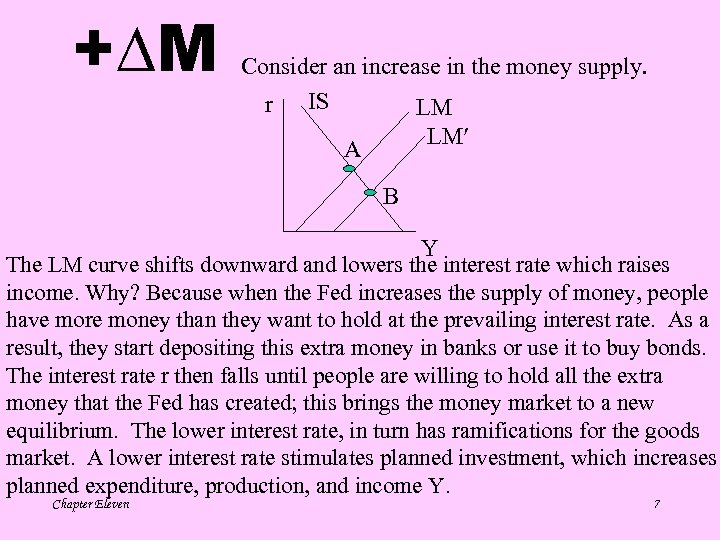+ M Consider an increase in the money supply. r IS LM LM A B Y The LM curve shifts downward and lowers the interest rate which raises income. Why? Because when the Fed increases the supply of money, people have more money than they want to hold at the prevailing interest rate. As a result, they start depositing this extra money in banks or use it to buy bonds. The interest rate r then falls until people are willing to hold all the extra money that the Fed has created; this brings the money market to a new equilibrium. The lower interest rate, in turn has ramifications for the goods market. A lower interest rate stimulates planned investment, which increases planned expenditure, production, and income Y. Chapter Eleven 7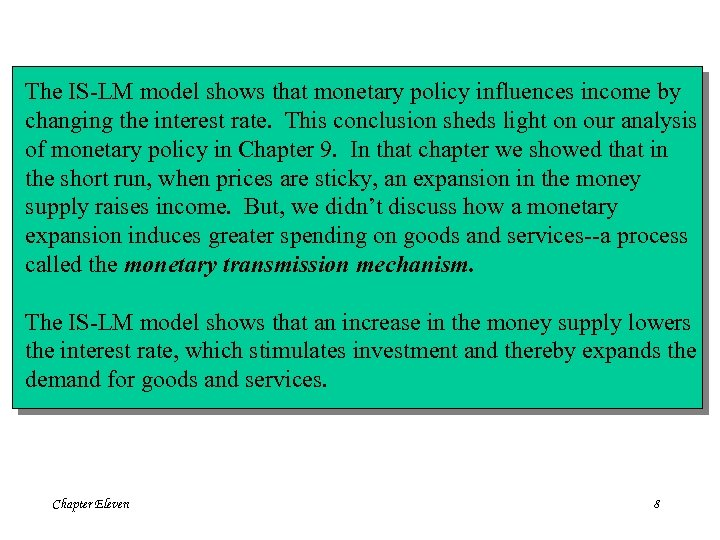The IS-LM model shows that monetary policy influences income by changing the interest rate. This conclusion sheds light on our analysis of monetary policy in Chapter 9. In that chapter we showed that in the short run, when prices are sticky, an expansion in the money supply raises income. But, we didn’t discuss how a monetary expansion induces greater spending on goods and services--a process called the monetary transmission mechanism. The IS-LM model shows that an increase in the money supply lowers the interest rate, which stimulates investment and thereby expands the demand for goods and services. Chapter Eleven 8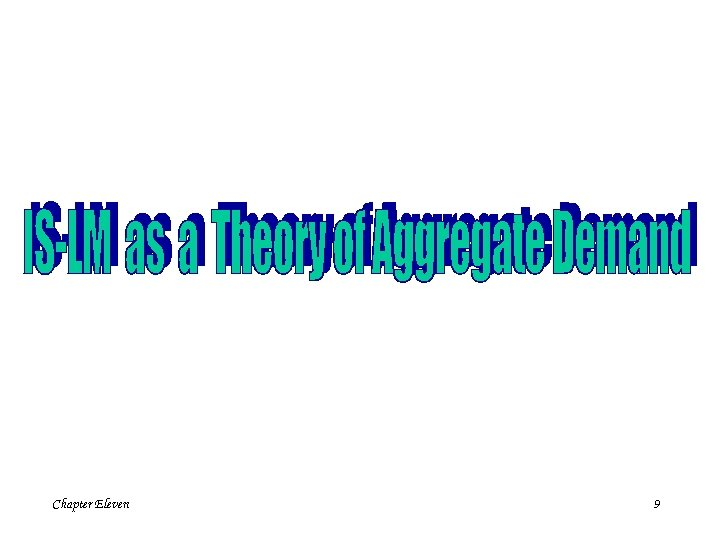Chapter Eleven 9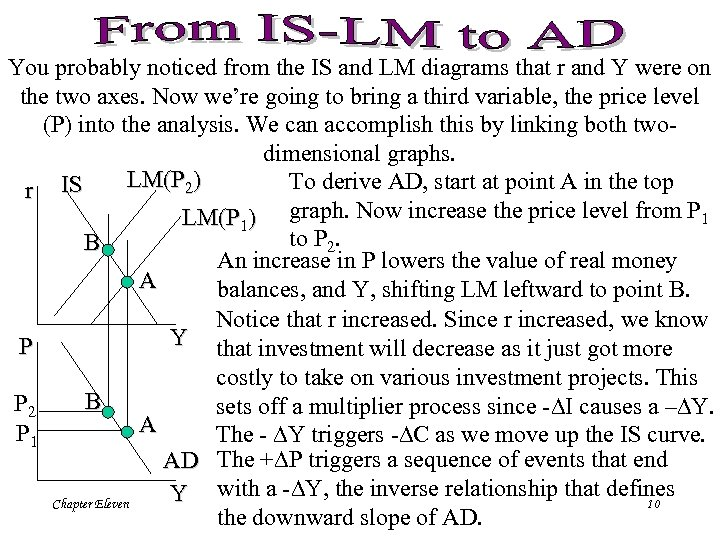You probably noticed from the IS and LM diagrams that r and Y were on the two axes. Now we’re going to bring a third variable, the price level (P) into the analysis. We can accomplish this by linking both twodimensional graphs. LM(P 2) To derive AD, start at point A in the top r IS LM(P 1) graph. Now increase the price level from P 1 to P 2. B An increase in P lowers the value of real money A balances, and Y, shifting LM leftward to point B. Notice that r increased. Since r increased, we know Y that investment will decrease as it just got more P costly to take on various investment projects. This B P 2 sets off a multiplier process since - I causes a – Y. A P 1 The - Y triggers - C as we move up the IS curve. AD The + P triggers a sequence of events that end Y with a - Y, the inverse relationship that defines Chapter Eleven 10 the downward slope of AD.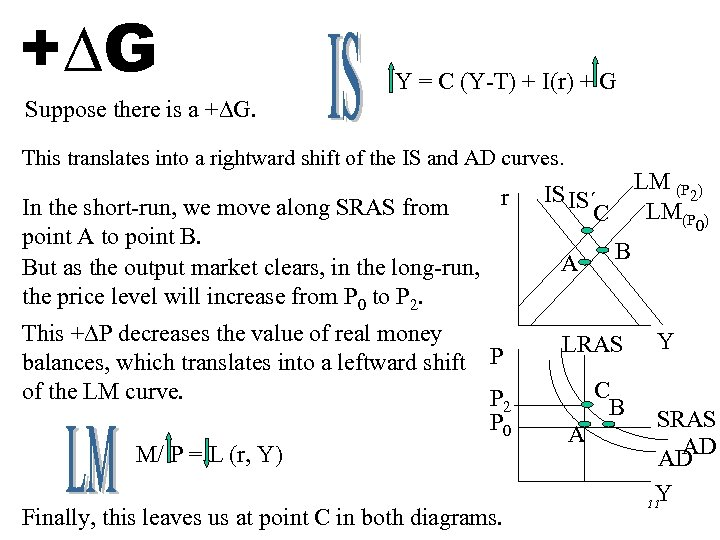+ G Y = C (Y-T) + I(r) + G Suppose there is a + G. This translates into a rightward shift of the IS and AD curves. r In the short-run, we move along SRAS from point A to point B. But as the output market clears, in the long-run, the price level will increase from P 0 to P 2. This + P decreases the value of real money balances, which translates into a leftward shift P of the LM curve. P 2 P 0 M/ P = L (r, Y) Chapter Eleven Finally, this leaves us at point C in both diagrams. LM (P ) 2 LM(P ) IS IS´ C A 0 B LRAS C B A Y SRAS AD´ AD Y 11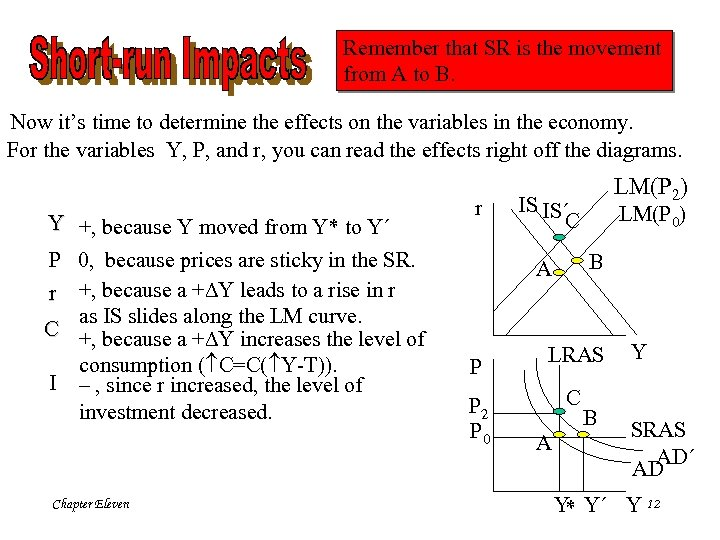Remember that SR is the movement from A to B. Now it’s time to determine the effects on the variables in the economy. For the variables Y, P, and r, you can read the effects right off the diagrams. Y +, because Y moved from Y* to Y´ P 0, because prices are sticky in the SR. r +, because a + Y leads to a rise in r as IS slides along the LM curve. C +, because a + Y increases the level of consumption ( C=C( Y-T)). I – , since r increased, the level of investment decreased. Chapter Eleven r IS IS´ C P 2 P 0 LM(P 0) B A P LM(P 2) LRAS C A B Y SRAS AD´ AD Y Y´ Y 12 *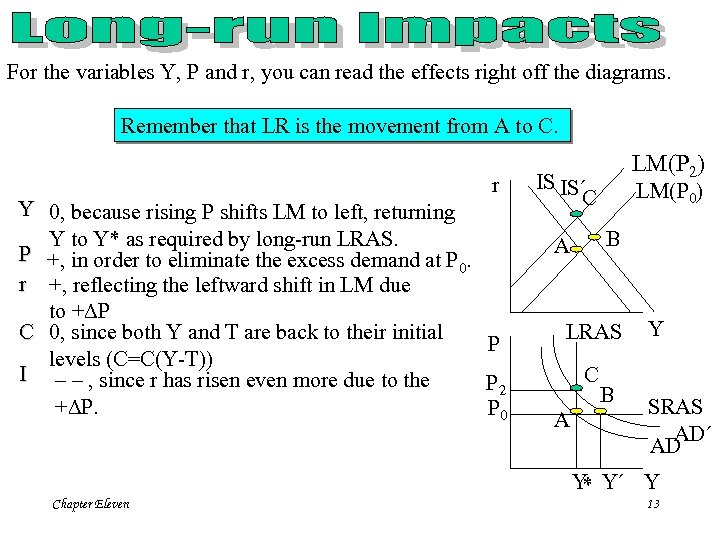For the variables Y, P and r, you can read the effects right off the diagrams. Remember that LR is the movement from A to C. r Y 0, because rising P shifts LM to left, returning Y to Y* as required by long-run LRAS. P +, in order to eliminate the excess demand at P. 0 r +, reflecting the leftward shift in LM due to + P C 0, since both Y and T are back to their initial P levels (C=C(Y-T)) I – – , since r has risen even more due to the P 2 + P. P 0 Chapter Eleven LM(P 2) IS IS´ C LM(P 0) B A LRAS C A B Y SRAS AD´ AD Y Y´ Y * 13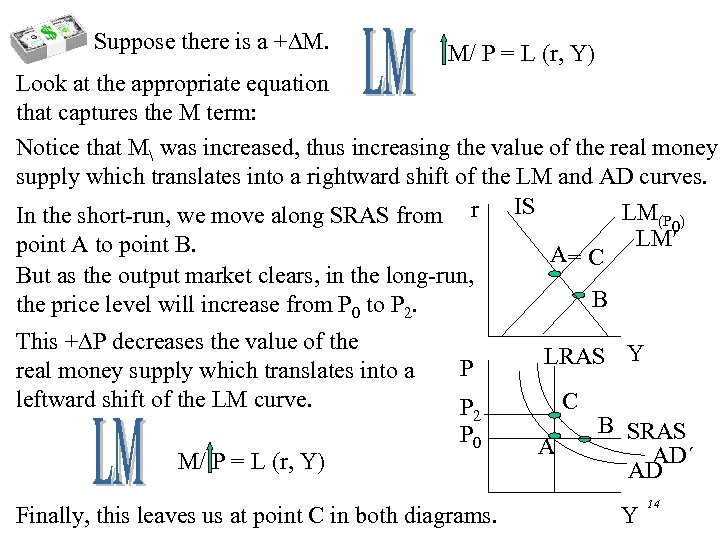Suppose there is a + M. M/ P = L (r, Y) Look at the appropriate equation that captures the M term: Notice that M was increased, thus increasing the value of the real money supply which translates into a rightward shift of the LM and AD curves. LM(P ) In the short-run, we move along SRAS from r IS 0 LM point A to point B. A= C But as the output market clears, in the long-run, B the price level will increase from P 0 to P 2. This + P decreases the value of the real money supply which translates into a leftward shift of the LM curve. M/ P = L (r, Y) Chapter Eleven P P 2 P 0 Finally, this leaves us at point C in both diagrams. LRAS Y C A B SRAS AD´ AD Y 14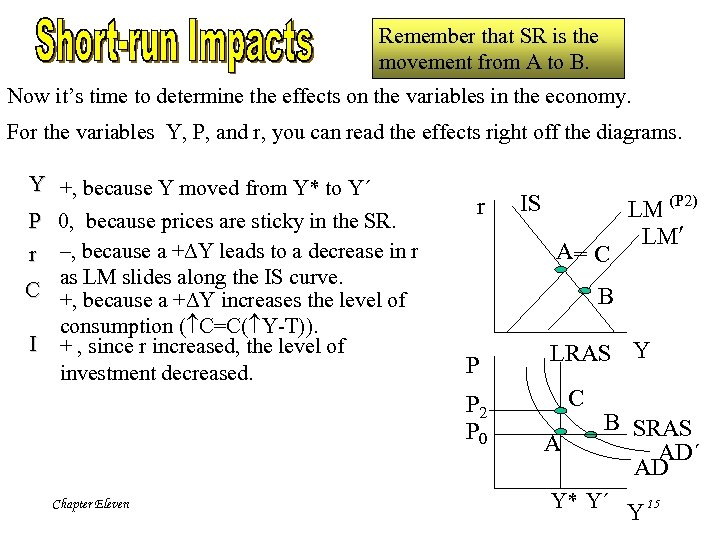Remember that SR is the movement from A to B. Now it’s time to determine the effects on the variables in the economy. For the variables Y, P, and r, you can read the effects right off the diagrams. Y +, because Y moved from Y* to Y´ P 0, because prices are sticky in the SR. r –, because a + Y leads to a decrease in r as LM slides along the IS curve. C +, because a + Y increases the level of consumption ( C=C( Y-T)). I + , since r increased, the level of investment decreased. r A= C (P 2) LM(P 0) LM B P P 2 P 0 Chapter Eleven IS LRAS Y C A B SRAS AD´ AD Y* Y´ Y 15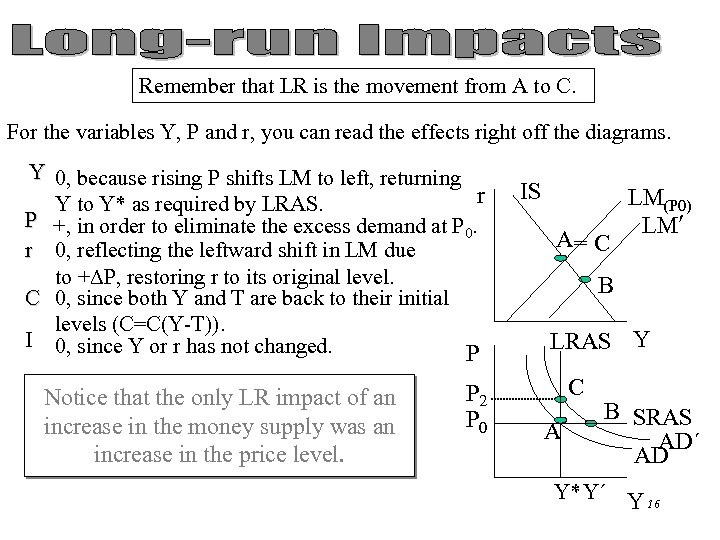Remember that LR is the movement from A to C. For the variables Y, P and r, you can read the effects right off the diagrams. Y 0, because rising P shifts LM to left, returning r Y to Y* as required by LRAS. P +, in order to eliminate the excess demand at P. 0 r 0, reflecting the leftward shift in LM due to + P, restoring r to its original level. C 0, since both Y and T are back to their initial levels (C=C(Y-T)). I 0, since Y or r has not changed. P Notice that the only LR impact of an increase in the money supply was an increase in the price level. Chapter Eleven P 2 P 0 IS A= C LM(P 0) LM B LRAS Y C A B SRAS AD´ AD Y* Y´ Y 16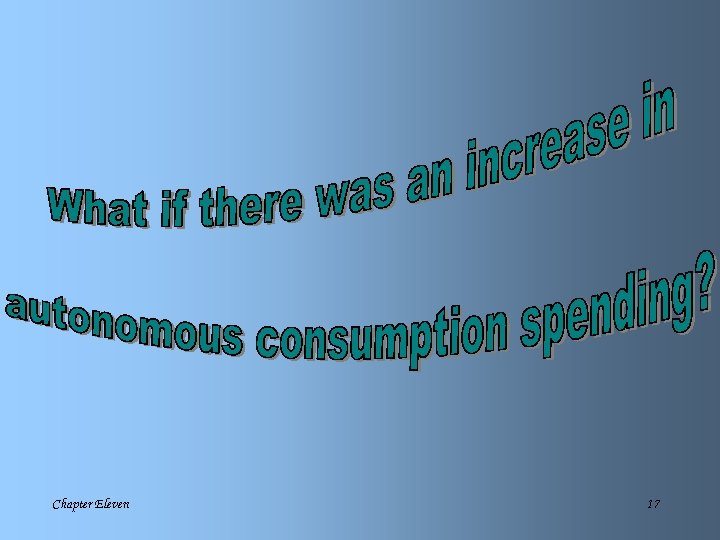Chapter Eleven 17C · B A P P 2 P 0 · · LRAS C · A · B · Chapter Eleven Y = C (Y-T) + I(r) + G 2) This leads to a rightward shift in AD to AD’. Short Run: Move from A to B. Long Run: Y Market clears at P 0 to P 2 from B to C. 3) + P causes LM(P 0) to shift leftward LRAS to LM(P 2) due to the lowering of the real value of the money supply. AD AD' Y M/ P = L (r, Y) -L M IS IS r LM(P 2) 1) + C causes the IS curve to shift LM(P 0) right to IS‘. IS' 18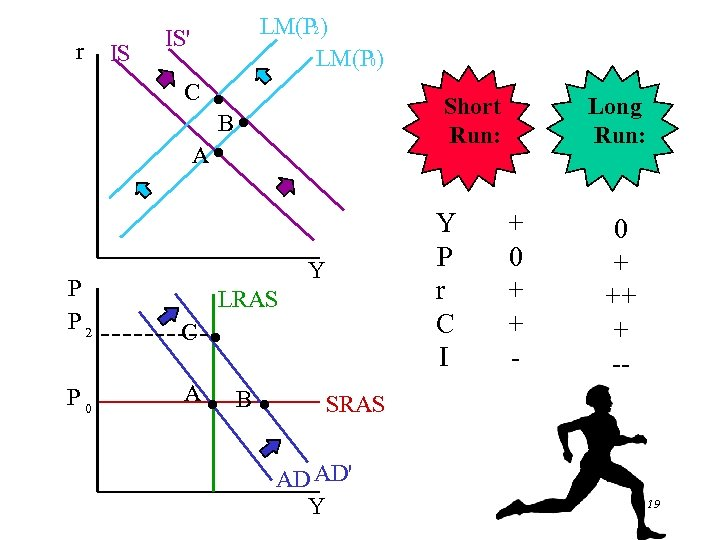r IS LM(P 2) LM(P 0) IS' C · B· A· Short Run: Y P r C I Y P P 2 C · P 0 A· B· Chapter Eleven LRAS Long Run: + 0 + + - 0 + ++ + -- SRAS AD AD' Y 19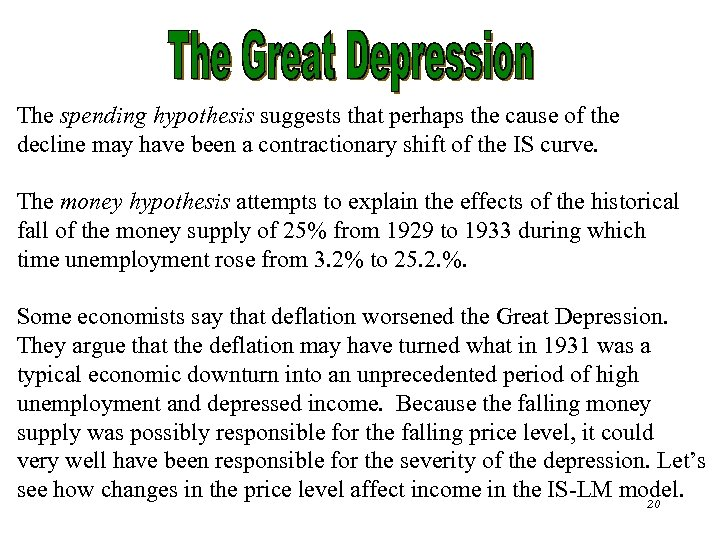The spending hypothesis suggests that perhaps the cause of the decline may have been a contractionary shift of the IS curve. The money hypothesis attempts to explain the effects of the historical fall of the money supply of 25% from 1929 to 1933 during which time unemployment rose from 3. 2% to 25. 2. %. Some economists say that deflation worsened the Great Depression. They argue that the deflation may have turned what in 1931 was a typical economic downturn into an unprecedented period of high unemployment and depressed income. Because the falling money supply was possibly responsible for the falling price level, it could very well have been responsible for the severity of the depression. Let’s see Chapter Eleven how changes in the price level affect income in the IS-LM model. 20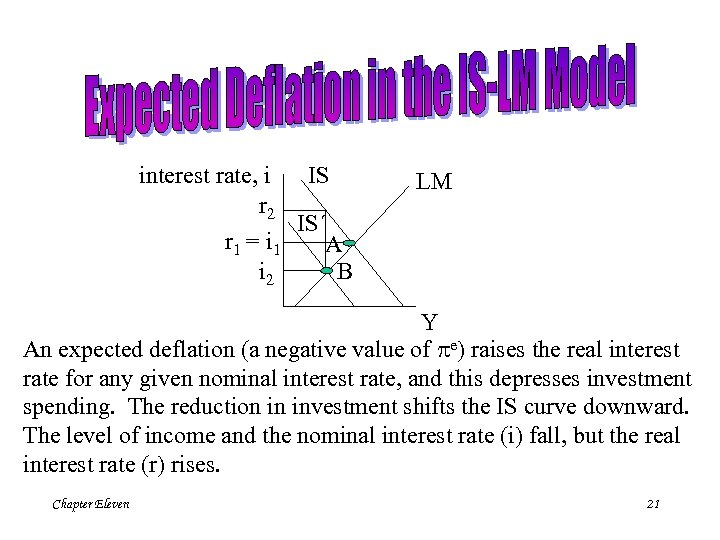IS interest rate, i r 2 IS´ r 1 = i 1 A i 2 B LM Y An expected deflation (a negative value of pe) raises the real interest rate for any given nominal interest rate, and this depresses investment spending. The reduction in investment shifts the IS curve downward. The level of income and the nominal interest rate (i) fall, but the real interest rate (r) rises. Chapter Eleven 21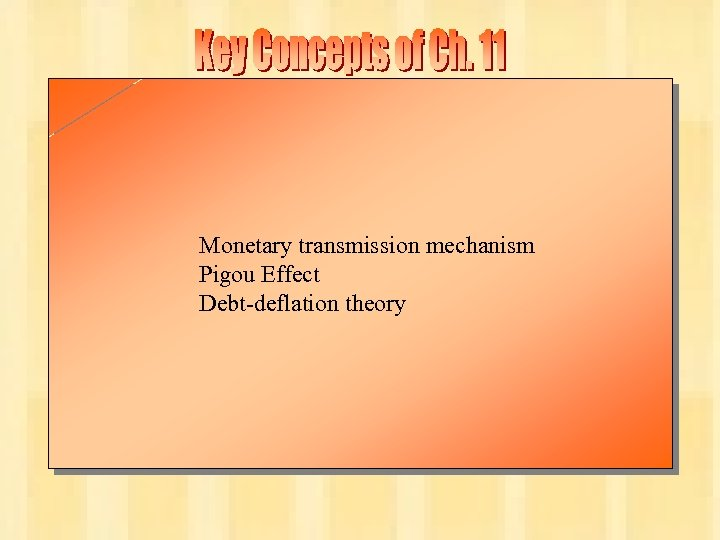Monetary transmission mechanism Pigou Effect Debt-deflation theory Chapter Eleven 22